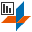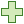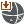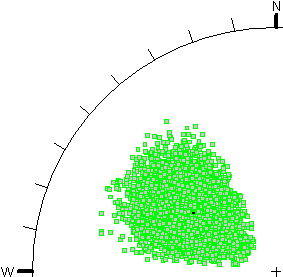# Probabilistic Joint Orientations

Select Statistics > Joint Orientationsto define the variability of Joint Orientations in the Joint Orientation Statistics dialog.

There are two methods of defining the variability of Joint Orientation in an UnWedge Probabilistic Analysis:

1. Orientation Definition Method = Dip / Dip Direction
2. Orientation Definition Method = Fisher Distribution

## Dip / Dip Direction

If you click the Addbutton for a particular joint property, then the Dip and Dip Direction of a Joint can each be assigned a statistical distribution, and are treated as independent random variables (i.e., during the statistical sampling, there is no correlation between the generated values of Dip and Dip Direction).

Select the desired joint tab from the left of the dialog and click the Addbutton in the Joint Orientation Statistics dialog to add Dip or Dip Direction as a random variable for a particular joint. You can assign a distribution type, mean, standard deviation, relative minimum, and relative maximum, for the variable.

The Dip / Dip Direction method allows you the flexibility to choose any two distributions for the Dip and Dip Direction. This can be useful if the actual distribution of orientations you are trying to model, is asymmetric (e.g., an elliptical distribution on the stereonet). However, if your orientation distribution is approximately circular, then you should use the Fisher Distribution option.

## Fisher Distribution

If you check the Use Fisher Distribution checkbox, then the Joint orientations will be randomly generated according to a Fisher Distribution. A Fisher Distribution assumes a symmetric distribution of 3-dimensional orientations around the mean, and only requires a single parameter (Fisher K value or Standard Deviation) to define the variability of the distribution.

The Fisher K value and the Standard Deviation of a Fisher distribution are related, so you can enter the standard deviation. In general, a Fisher Distribution is recommended for generating random joint plane orientations, because it provides more predictable orientation distributions, and lessens the chance of input data errors.

Select the desired joint tab from the left of the dialog and check the Use Fisher Distribution box at the top of the dialog to assign a Fisher Distribution to a particular joint.

## Import from Dips

If the Orientation Definition Method = Fisher Distribution, then you can import the Joint mean orientation and Fisher K value from a Dips file, by selecting the Import from Dipsbutton and selecting the desired joint set from the file.

## Comparison of Dip/Dip Direction and Fisher Distribution Methods

The following figures illustrate an example of the difference between the two methods of generating random plane orientations in UnWedge.

• In Figure A, the Dip and Dip Direction are specified as independent random variables, using a Normal distribution and a standard deviation of 10 degrees for both dip and dip direction.
• In Figure B, a Fisher Distribution is used, with a standard deviation of 10 degrees.

In both cases, 10,000 random plane orientations were generated. The stereonet projection method is Equal Angle.Figure A - independent sampling of Dip and Dip DirectionFigure B - Fisher distribution

As you can see, the Fisher Distribution results in an approximately circular distribution of orientations (poles) around the mean orientation (on an Equal Angle stereonet projection). The Dip/Dip Direction method results in an asymmetric oval-shaped distribution of poles, for the given input parameters.

The advantage of the Fisher Distribution is the ability to produce a symmetric distribution of orientations around the mean orientation, for 3-dimensional orientation data, regardless of the direction of the mean orientation. In general, the Fisher Distribution is recommended over the Dip/Dip Direction (two-variable) approach, because the data input is simpler, and the results are more reliable [ Meyers and Priest (2000)].

If your actual joint set pole distribution is asymmetric, then you can use the Dip/Dip Direction method to specify independent distributions for the Dip and Dip Direction.